Manfred MOHRHalf Planes (1995-1998)

This work-phase (1995-97) is based on the 6-D hypercube. As indicated for the Laserglyphs (1991), this complex structure has 32 diagonals from which 23040 "diagonal-paths" can be calculated combinatorially.

A random selecton of two "diagonal-paths" from this alphabet of signs provides the building blocks for each work in this work-phase. Both "diagonal-paths" are shown here as thick white lines.

A six-dimensional "diagonal-path" is built from six consecutive connected vectors, each having a different but distinct direction. Each direction represents one of the six mathematical dimensions.

All six vectors of one "diagonal-path" are matched with there corresponding vector on the other "diagonal-path", resulting in six vector pairs. Each vector pair is oriented to form a non-intersecting planar quadrilateral, and then is completed visually with thin lines. Thus, six rectangles are created, and together with the two "diagonal-paths", describe a contour line, resulting in the most surprising shapes.

The shapes of the b/w drawings and grey acrylic paintings permit the viewer to experience 6-D space, which is beyond mental representation, but nonetheless calculatable.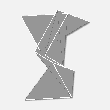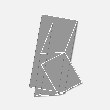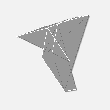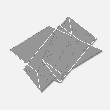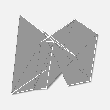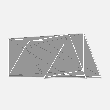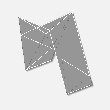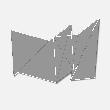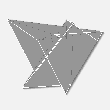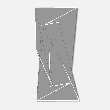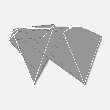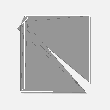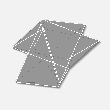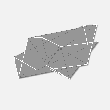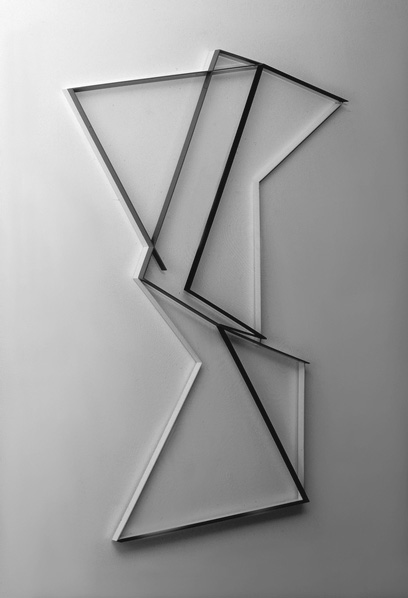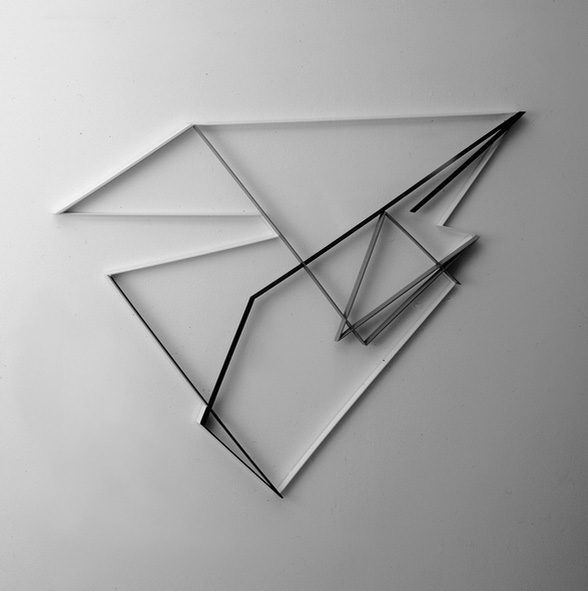Metal reliefs 1997-1998